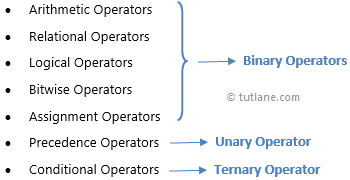# C# Operators (Arithmetic, Relational, Logical, Assignment, Precedence)

In c#, the Operator is a programming element that specifies what kind of operation needs to perform on operands or variables. For example, an addition (+) operator in c# is used to perform the sum operation on operands.

In c#, we have a different type of operators available, those areThese operators are categorized as a different group such as Binary Operators, Unary Operators and Ternary Operators based on taking the number operands to perform the operations.

Suppose, if the operator takes one operand to perform the operation such as precedence operator (++), then those will call as Unary Operators. In case, if the operator takes two operands to perform the operation such as arithmetic operator (-, +, *, /), then those will call as a Binary Operators and we have a Ternary Operator that takes three operands to perform the operation, such as conditional operator (?:).

Now we will learn each operator in a detailed manner with examples in c# programming language.

## C# Arithmetic Operators

In c#, Arithmetic Operators are useful to perform basic arithmetic calculations like addition, subtraction, division, etc. based on our requirements.

To know more about Arithmetic Operators, check this C# Arithmetic Operators with Examples.

## C# Relational Operators

In c#, Relational Operators are useful to check the relation between two operands like we can determine whether two operand values equal or not, etc. based on our requirements.

To know more about Relational Operators, check this C# Relational Operators with Examples.

## C# Logical Operators

In c#, Logical Operators are useful to perform the logical operation between two operands like AND, OR and NOT based on our requirements and the operands must contain only Boolean values otherwise Logical Operators will throw an error.

To know more about Logical Operators, check this C# Logical Operators with Examples.

## C# Bitwise Operators

In c#, Bitwise Operators will work on bits and these are useful to perform the bit by bit operations such as Bitwise AND (&), Bitwise OR (|), Bitwise Exclusive OR (^), etc. on operands and we can perform bit-level operations on Boolean and integer data.

To know more about Bitwise Operators, check this C# Bitwise Operators with Examples.

## C# Assignment Operators

In c#, Assignment Operators are useful to assign a new value to the operand and these operators will work with only one operand.

To know more about Assignment Operators, check this C# Assignment Operators with Examples.

## C# Operators Precedence

In c#, Operators Precedence is useful to define multiple operators in single expression and the evaluation of expression can be happened based on the priority of operators.

To know more about the Precedence of Operators, check this C# Operators Precedence with Examples.